Sec. 4.8 concluded with a table of factors for the contributions to coeﬃcients of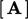and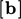for the ﬁxed value and ﬁxed gradient boundary conditions.

It distinguishes between contributions for the discretisation of an advection term which requires values at faces, and the Laplacian term which requires the normal gradient.

A mixed ﬁxed value/gradient condition is deﬁned by introducing a value fraction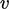for which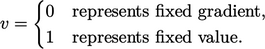(4.9)
Within the range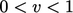the condition operates in between ﬁxed value and ﬁxed gradient. The mixed condition is simply implemented by blending the ﬁxed value and ﬁxed gradient contributions by, as shown in the table below.

 factor mixed value internal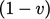value boundary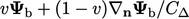gradient internal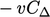gradient boundary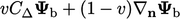This mixed condition provides the framework for a boundary condition that can switch between a ﬁxed value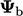and ﬁxed gradient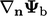, by changing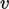. Switching is often based on ﬂow direction,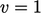corresponding to inﬂow and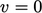to outﬂow.

Some boundary conditions can operate in the range of value fractions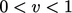. The Robin condition, described next, can also be expressed as a mixed condition with varying.

### Robin condition

The Robin condition5 combines the value and normal gradient at the boundary through an expression: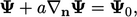(4.10)
where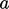is a scalar coeﬃcient with units of length and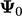is some constant value of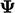.

The Robin condition can be treated like the mixed condition by relating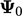to a reference ﬁxed value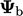and gradient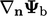at a boundary, according to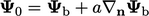.

Substituting for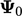in Eq. (4.10) and making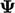the subject of the equation gives: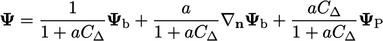Comparison with the value factors in the previous table shows that the Robin condition corresponds to the mixed condition with the value fraction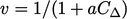.

In this form,and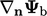relate to the limits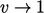and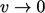, respectively. Values can be selected in these limits to represent the physics of the condition.

In many cases the reference gradient is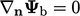such that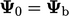in Eq. (4.10 ). For example a condition for temperature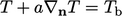would tend to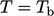as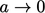and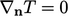as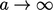.

The value fraction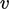includes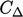, so the condition operates “in the middle” between the ﬁxed value and gradient when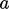is the same order of magnitude as the boundary cell height.

5after Victor Robin (1855-1897).

Notes on CFD: General Principles - 4.9 Mixed ﬁxed value/gradient